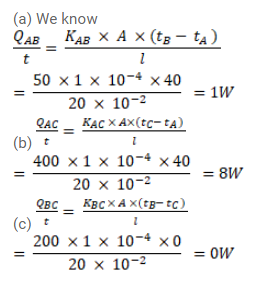# Three rods of lengths 20cm each and area of cross - section

Question:

Three rods of lengths $20 \mathrm{~cm}$ each and area of cross - section $1 \mathrm{~cm}^{2}$ are joined to form a triangle $A B C$. The conductivities of the rods are $\mathrm{K}_{\mathrm{AC}}=50 \mathrm{~J} / \mathrm{m}-\mathrm{s}-{ }^{\circ} \mathrm{C}, \mathrm{K}_{\mathrm{BC}}=200 \mathrm{~J} / \mathrm{m}-\mathrm{s}-{ }^{\circ} \mathrm{C}$ and $\mathrm{K}_{\mathrm{AC}}=400 \mathrm{~J} / \mathrm{m}-\mathrm{s}-{ }^{\circ} \mathrm{C}$. Then junctions $\mathrm{A}, \mathrm{B}$ and $\mathrm{C}$ are maintained at $40^{\circ} \mathrm{C}, 80^{\circ} \mathrm{C}$ and $80^{\circ} \mathrm{C}$ respectively. Find the rate of heat flowing through the rods $A B, A C$ and $B C$.

Solution: